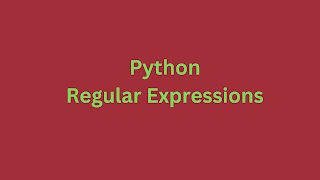### Python Regex: The 5 Exclusive ExamplesRegular expressions (regex) are powerful tools for pattern matching and text manipulation in Python. Here are five Python regex examples with explanations: 01 Matching a Simple Pattern import re text = "Hello, World!" pattern = r"Hello" result = re.search(pattern, text) if result:     print("Pattern found:", result.group()) Output: Output: Pattern found: Hello This example searches for the pattern "Hello" in the text and prints it when found. 02 Matching Multiple Patterns import re text = "The quick brown fox jumps over the lazy dog." patterns = [r"fox", r"dog"] for pattern in patterns:     if re.search(pattern, text):         print(f"Pattern '{pattern}' found.") Output: Pattern 'fox' found. Pattern 'dog' found. It searches for both "fox" and "dog" patterns in the text and prints when they are found. 03 Matching Any Digit   import re text = "The price of the

# How to Convert Dictionary to Dataframe: Pandas from_dict

Pandas is a data analysis Python library.  The example shows you to convert a dictionary to a data frame. The point to note here is DataFrame will take only 2D data. So you need to supply 2D data.

## Pandas Dictionary to Dataframe

import pandas as pd

import numpy as np

data_dict = {'item1' : np.random.randn(4), 'item2' : np.random.randn(4)}

df3=pd.DataFrame.from_dict(data_dict, orient='index')

print(df3)

Output

0 1 2 3 item1 -0.109300 -0.483624 0.375838 1.248651 item2 -0.274944 -0.857318 -1.203718 -0.061941

Explanation

Using the NumPy package, created a dictionary with random values. There are two items - item 1 and item 2. The data_dict is input to the data frame. The from_dict method needs two parameters. These are data_dict and index. Here's the syntax you can refer to quickly.

Related